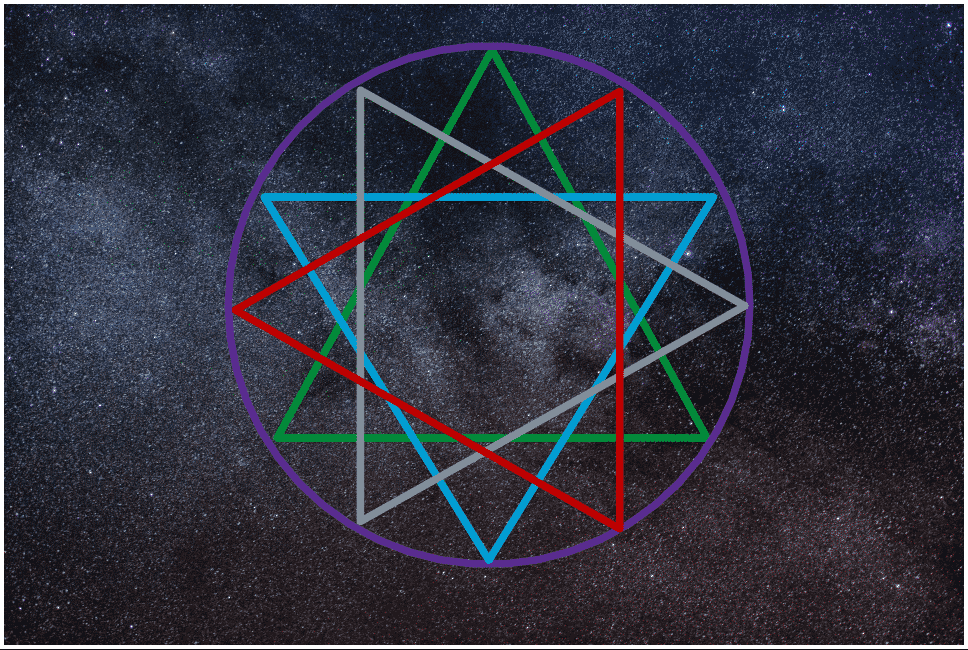Urania’s code holds the balance that is the Way of the Goddess in the divine dodecagram.  The dodecagram reduces to three, and three is a magic number.  Three holds all that there is and all that we need to know.  It is representative of and a constant reminder of our origins.  One is the Goddess, two is the God, and three is the well of souls born of their union.  Three is the zero-point field, the force, all that ever was and all that ever will be, the field that all consciousness is born into and originates from.

• The dodecagram is made of four triangles, that represent the four sacred elements–fire, water, air, and earth.
• The dodecagram is two hexagrams intertwined.
• One hexagram consists of Fire and Air.
• The other hexagram consists of Earth and Water.
• The four intersecting triangles result in 12 points placed exactly 360 degrees apart to form a perfect circle.
• The three points of each triangle points to a direction.
• The first point of each triangle points to a cardinal direction.
• The second point of each triangle points to a primary intercardinal direction.
• The third point of each triangle points to a secondary intercardinal direction.

1. East
2. South
3. West
4. North

1. Fire
2. Water
3. Air
4. Earth